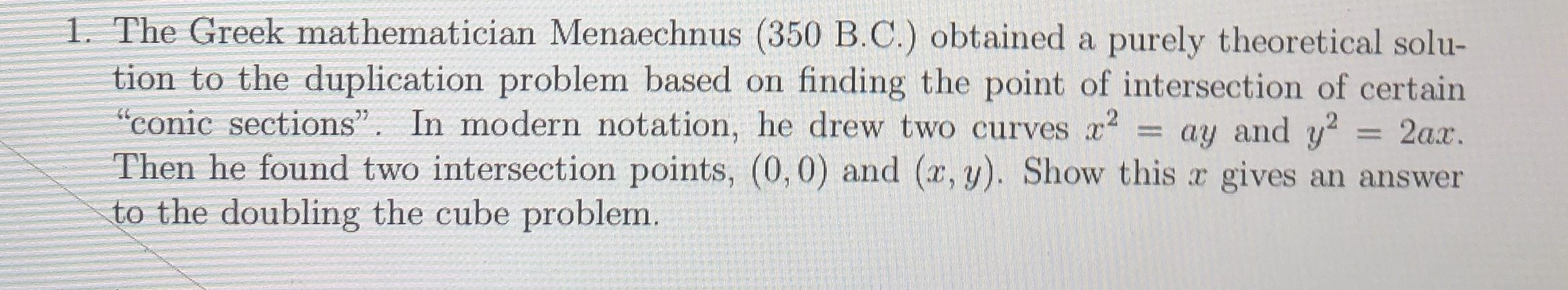# 1. The Greek mathematician Menaechnus (350 B.C.) obtained a purely theoretical solu-tion to the duplication problem based on"conic sections". In modern notation, he drew two curves x2Then he found two intersection points, (0,0) and (x,y). Show this x gives an answerto the doubling the cube problem.finding the point of intersection of certainay and y22ax.

Question
15 viewshelp_outlineImage Transcriptionclose1. The Greek mathematician Menaechnus (350 B.C.) obtained a purely theoretical solu- tion to the duplication problem based on "conic sections". In modern notation, he drew two curves x2 Then he found two intersection points, (0,0) and (x,y). Show this x gives an answer to the doubling the cube problem. finding the point of intersection of certain ay and y2 2ax. fullscreen
check_circle

Step 1

To justify Manaechnus's geometric method to solve "dublication of cube" problem

Step 2

The duplication of cube problem consists in finding a solution (algebraic or geometric) to the equation z^3 = 2a^3; ...

### Want to see the full answer?

See Solution

#### Want to see this answer and more?

Solutions are written by subject experts who are available 24/7. Questions are typically answered within 1 hour.*

See Solution
*Response times may vary by subject and question.
Tagged in

### Math